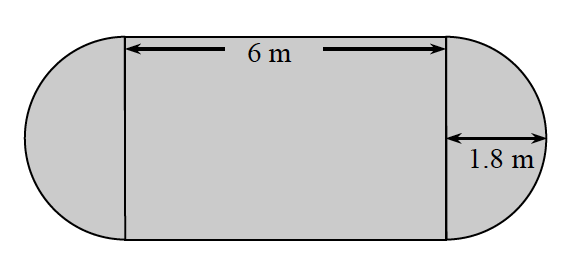Home > ACC7 > Chapter cc33 > Lesson cc33.2.5 > Problem3-116

3-116.

Find the area and perimeter of the shape below. Show your work. Homework Help ✎Refer to the Math Notes box in Lesson 3.2.2 for review on circle area and circumference.

Since the radius of the circle is $1.8$ m, what is the diameter?

$3.6$ m
Now that you know the diameter, what is the width of the rectangle?

Now find the area of each separate shape.
Circle: $A=\pi·r^2$
Rectangle: (length)(width)

The total area of the shape is about $31.78\text{ m}^2$.
What is the perimeter?